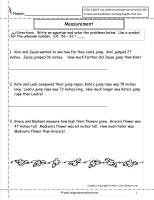﻿ CCSS 2.MD.5 Worksheets, Comparing Measurements Worksheets

# Common Core State Standard 2.MD.5

## Measurement & Data

### Relate addition and subtraction to length.

Use addition and subtraction within 100 to solve word problems involving lengths that are given in the same units, e.g., by using drawings (such as drawings of rulers) and equations with a symbol for the unknown number to represent the problem.

###Compare Measurements

Common Core State Standards: 2.MD.5
Measurement & Data
Relate addition and subtraction to length.

Use addition and subtraction within 100 to solve word problems involving lengths that are given in the same units, e.g., by using drawings (such as drawings of rulers) and equations with a symbol for the unknown number to represent the problem.